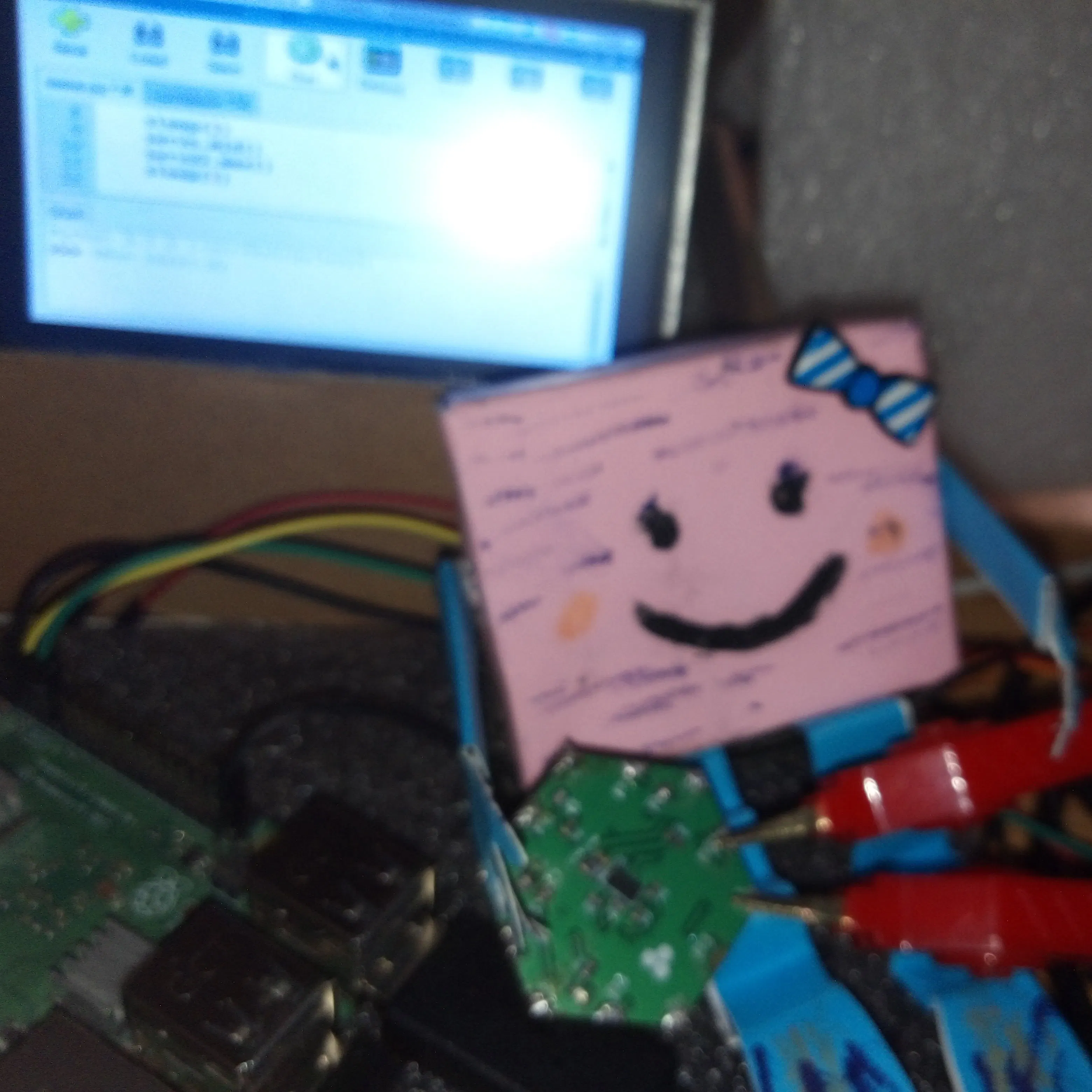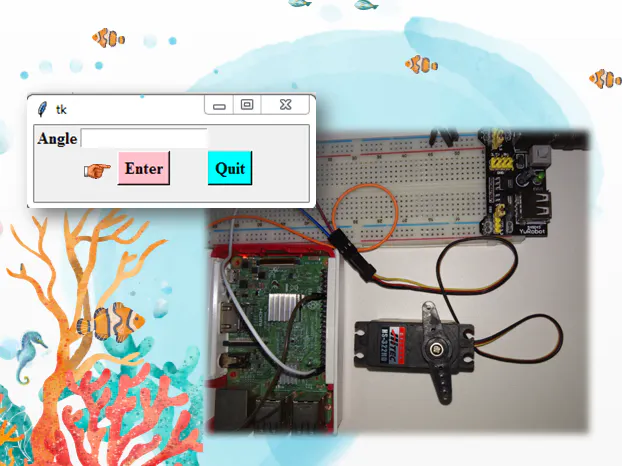Published

# Controlling a Servo Motor with Raspberry Pi and Python GUI

In this tutorial we are going present how to precisely control a servo using the Raspberry Pi and Python TK GUI.

IntermediateFull instructions provided1 hour7,969## Things used in this project

### Hardware componentsRaspberry Pi 3 Model B
×1
×1×1Jumper wires (generic)
×1

## Code

### The code in python 3

Python
```from tkinter import *
#Thinter in python 2
import RPi.GPIO as io
import time
io.setmode(io.BOARD)
io.setup(11,io.OUT)
p=io.PWM(11,50)
p.start(2.5)
root = Tk()
Label(root, text="Angle").grid(row=0)
e1 = Entry(root)
e1.grid(row=0, column=1)

def cal():
global dc
deg1 =e1.get()
deg = abs(float(deg1))
dc = 0.056*deg + 2.5
p.ChangeDutyCycle(dc)
print(deg, dc)

b1= Button(root, text = "Enter",bg="pink", command =cal)
b1.grid(row=2, column=1)
b3 = Button(root, text='Quit', bg= "cyan", command=root.quit)
b3.grid(row=2, column=3)
root.mainloop()

```

## Credits

### Aula Jazmati

27 projects • 95 followers
💡